# RD Sharma Solutions for Class 6 Maths Chapter 22: Data Handling - II (Pictographs)

After collection and organization of the given set of data, the next step is to get some useful information from it. So the data should be represented pictorially. The study of numerical data through pictures or graphs is known as the pictorial representation or graph of the data. The method of representing numerical data by using picture symbols is called a pictograph.

The primary objective of designing solutions is to help students understand the concepts in a better way. The students can make use of solutions’ PDF while solving the problems as per RD Sharma textbook. To gain expertise in the subject, the students can download RD Sharma Solutions for Class 6 Maths Chapter 22 Data Handling – II (Pictographs) PDF which are provided here.

## RD Sharma Solutions for Class 6 Maths Chapter 22: Data Handling – II (Pictographs) Download PDF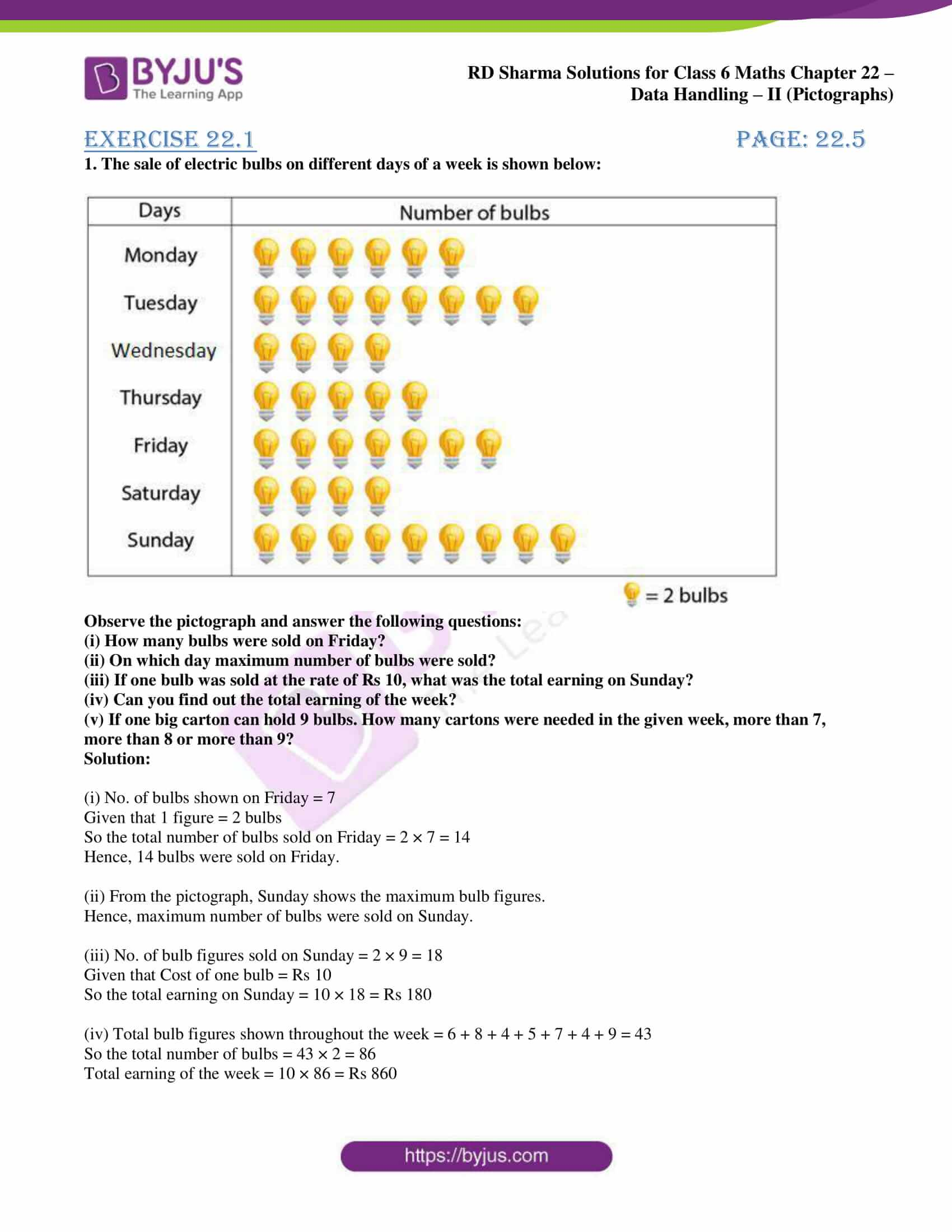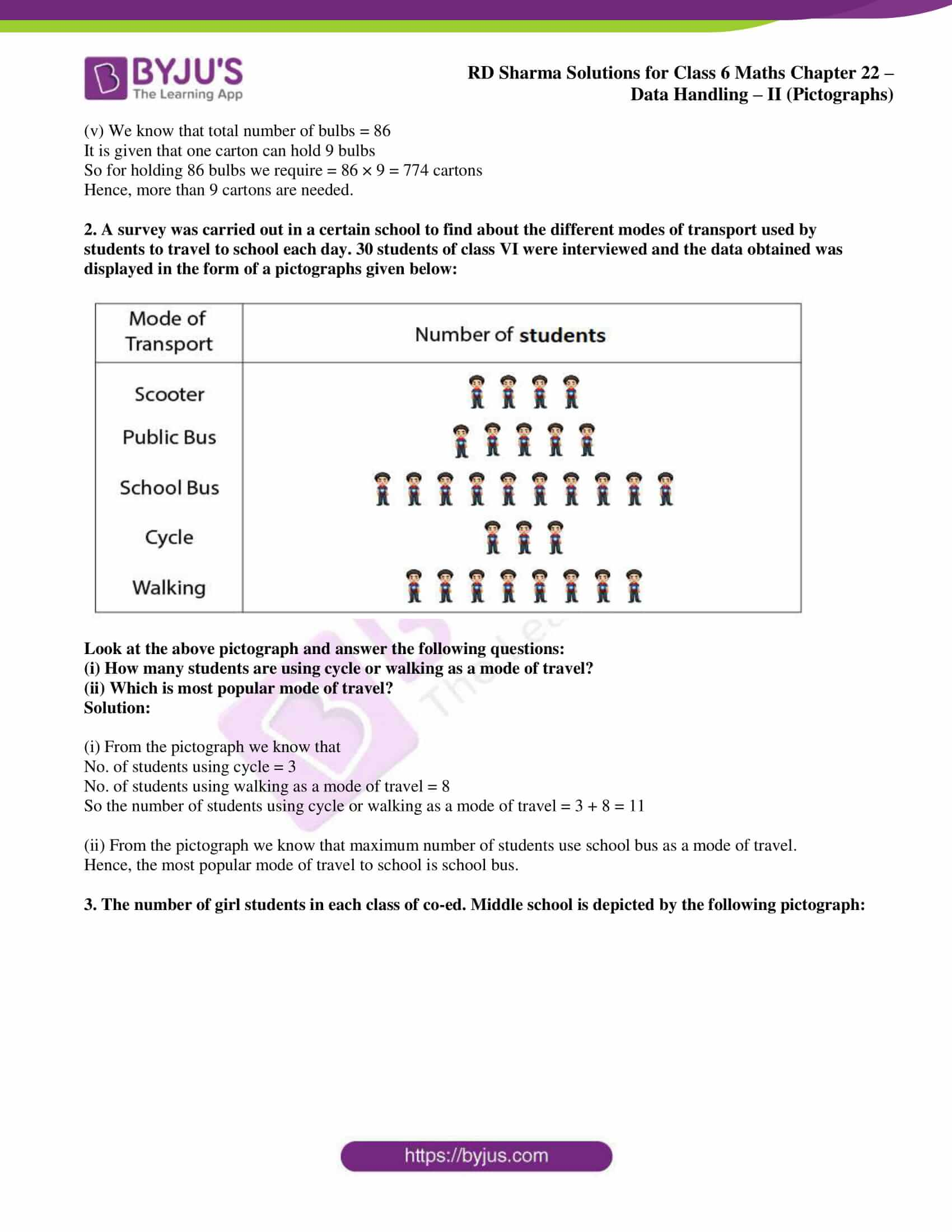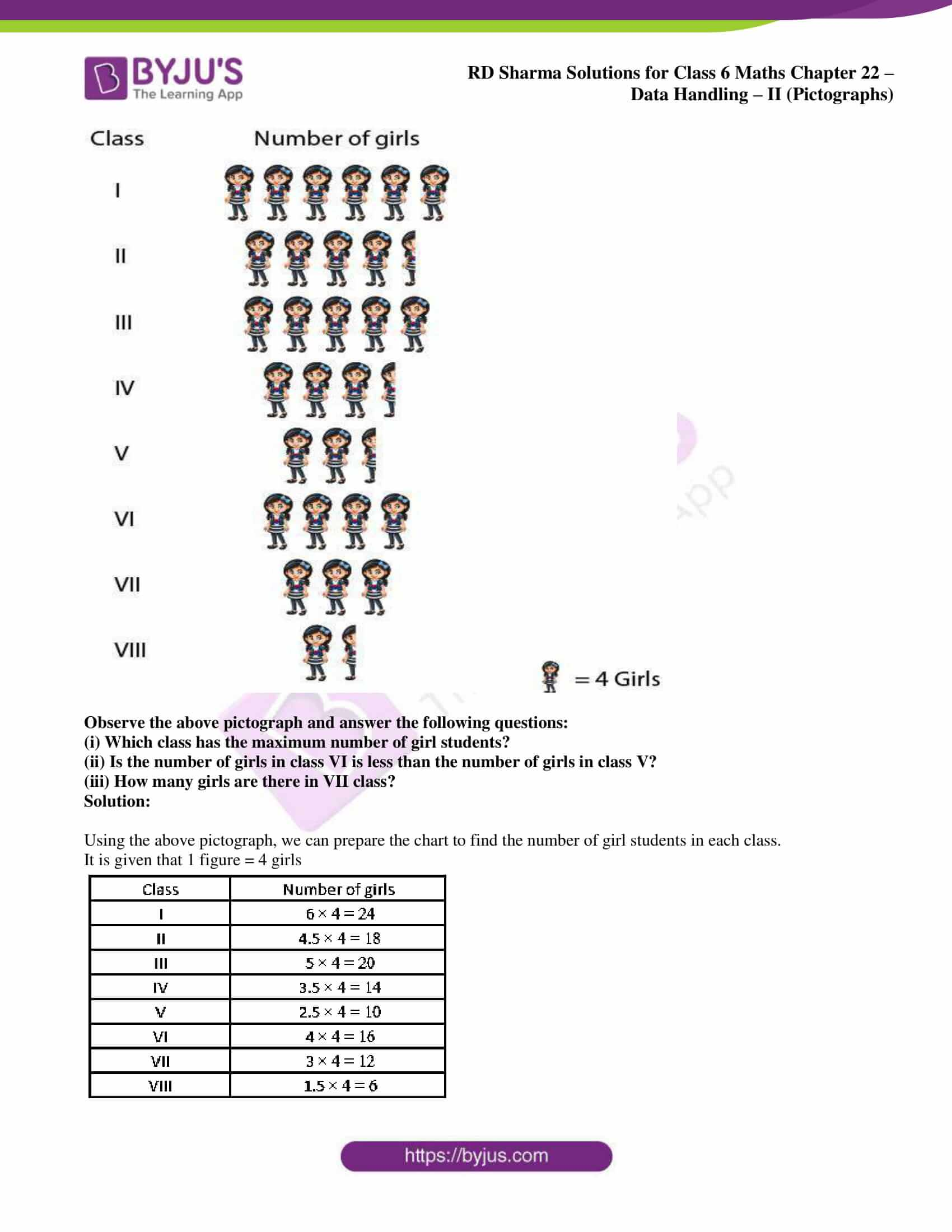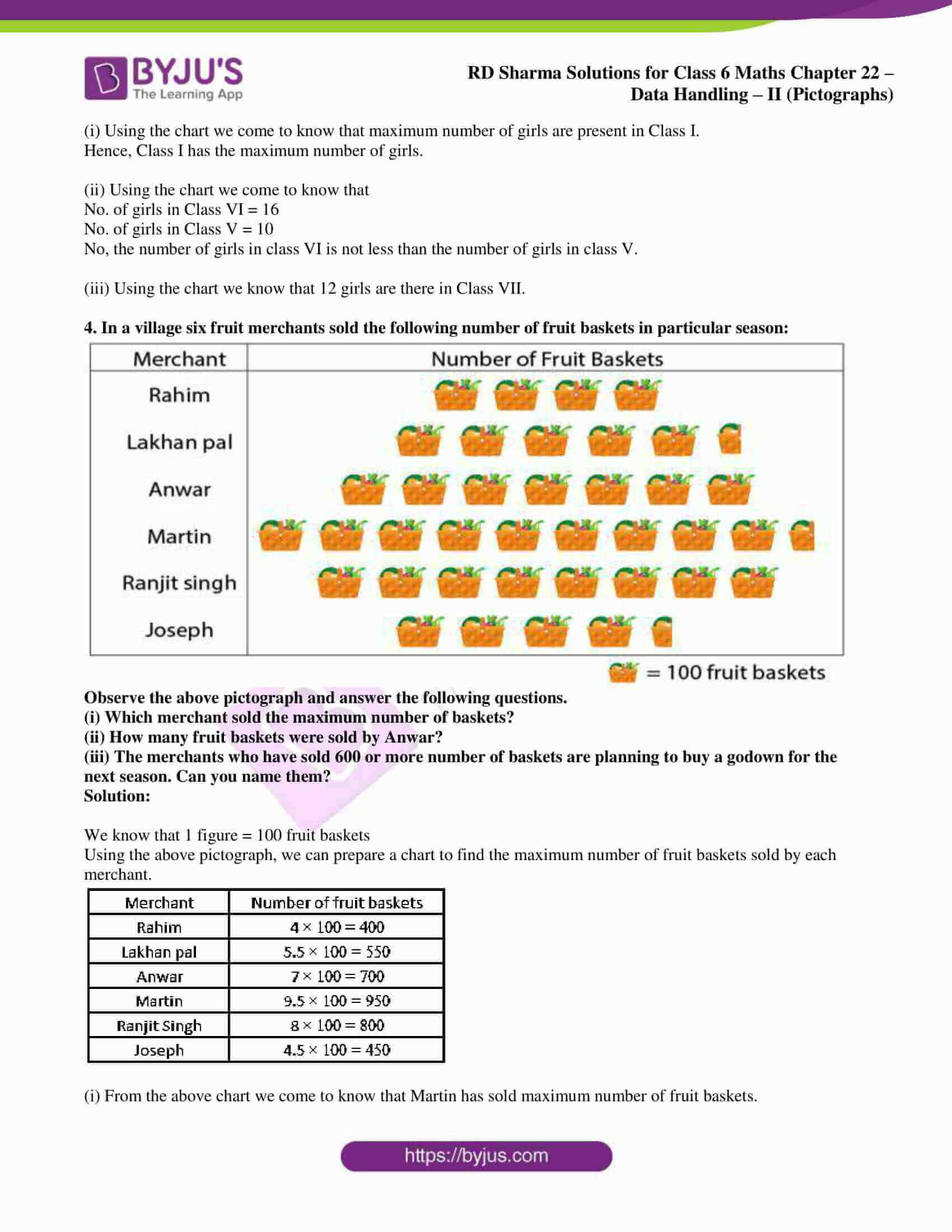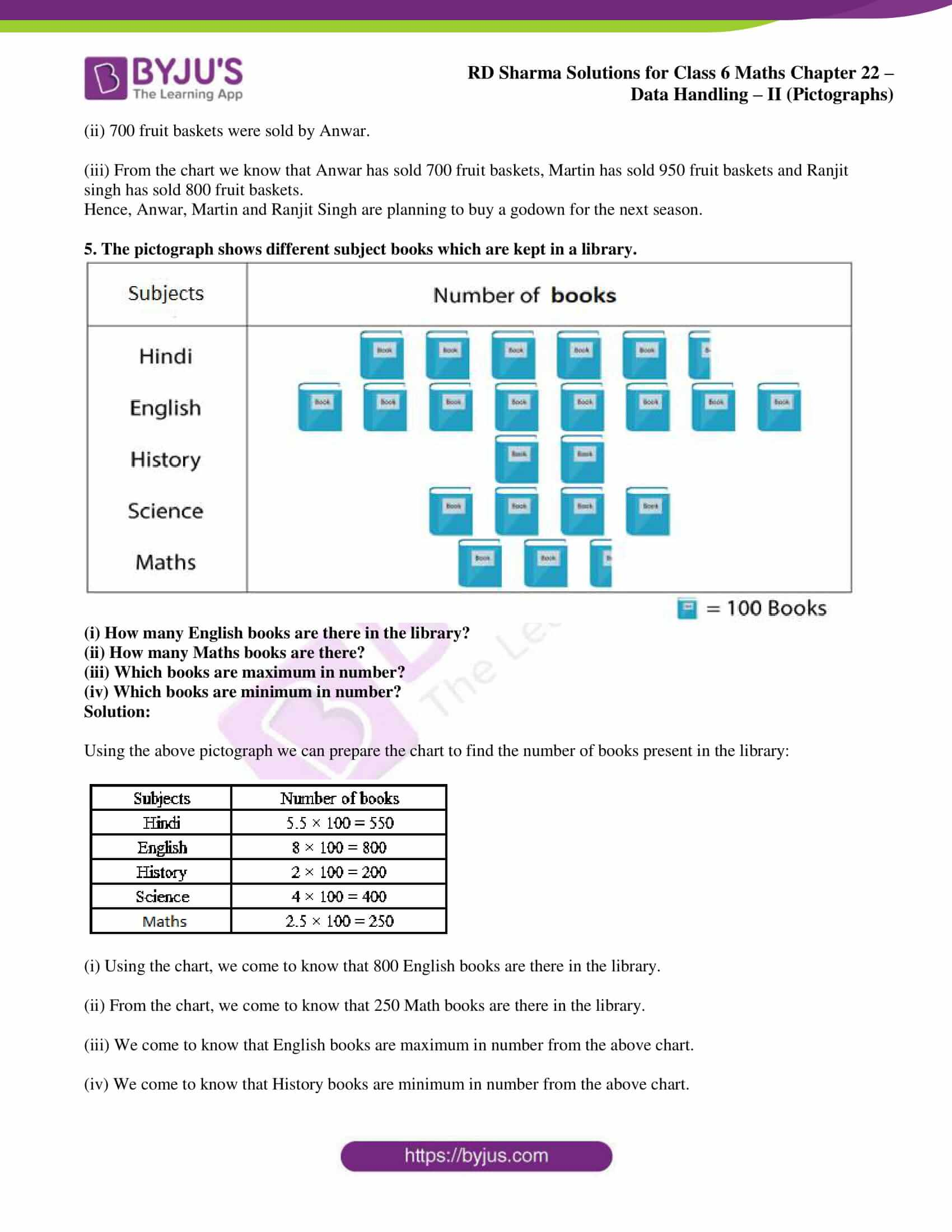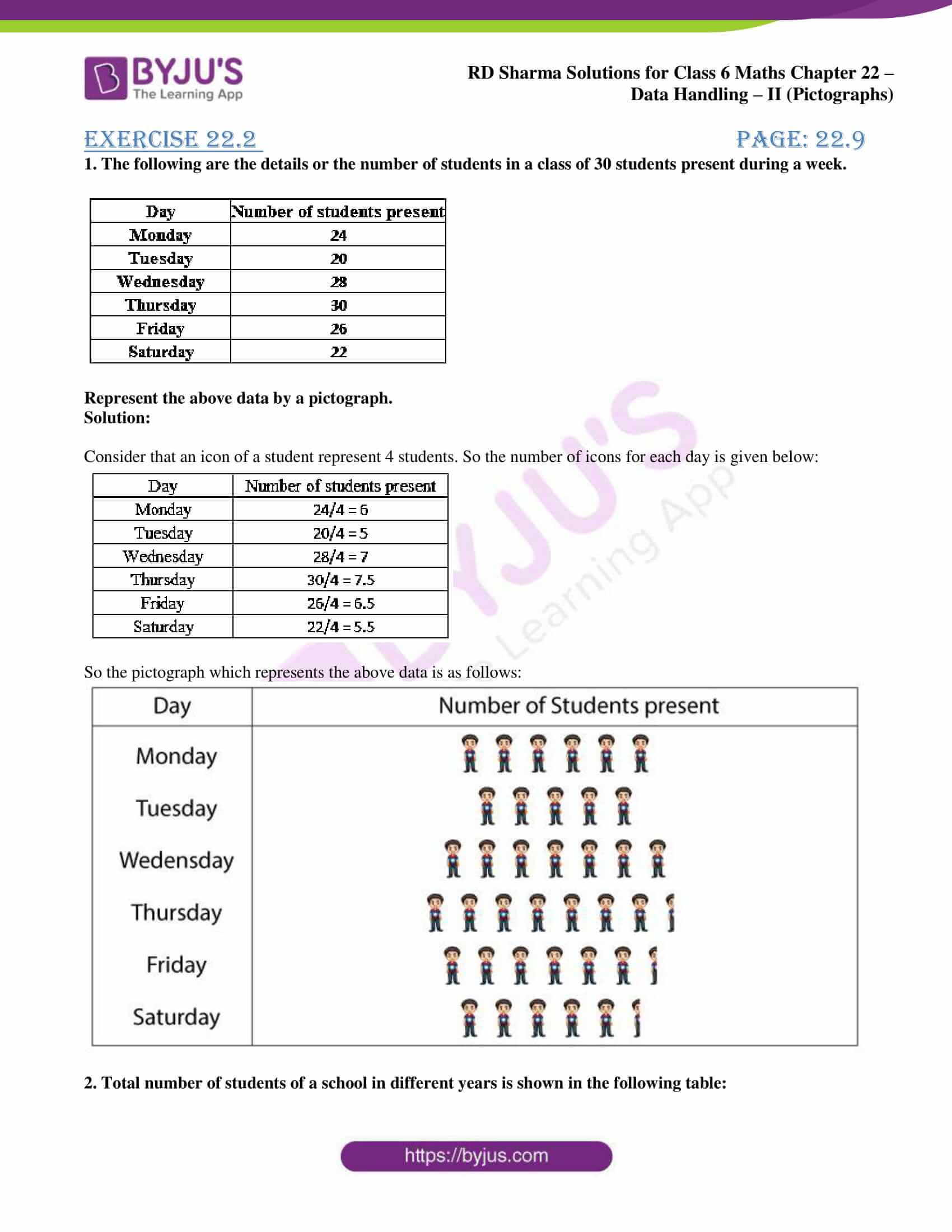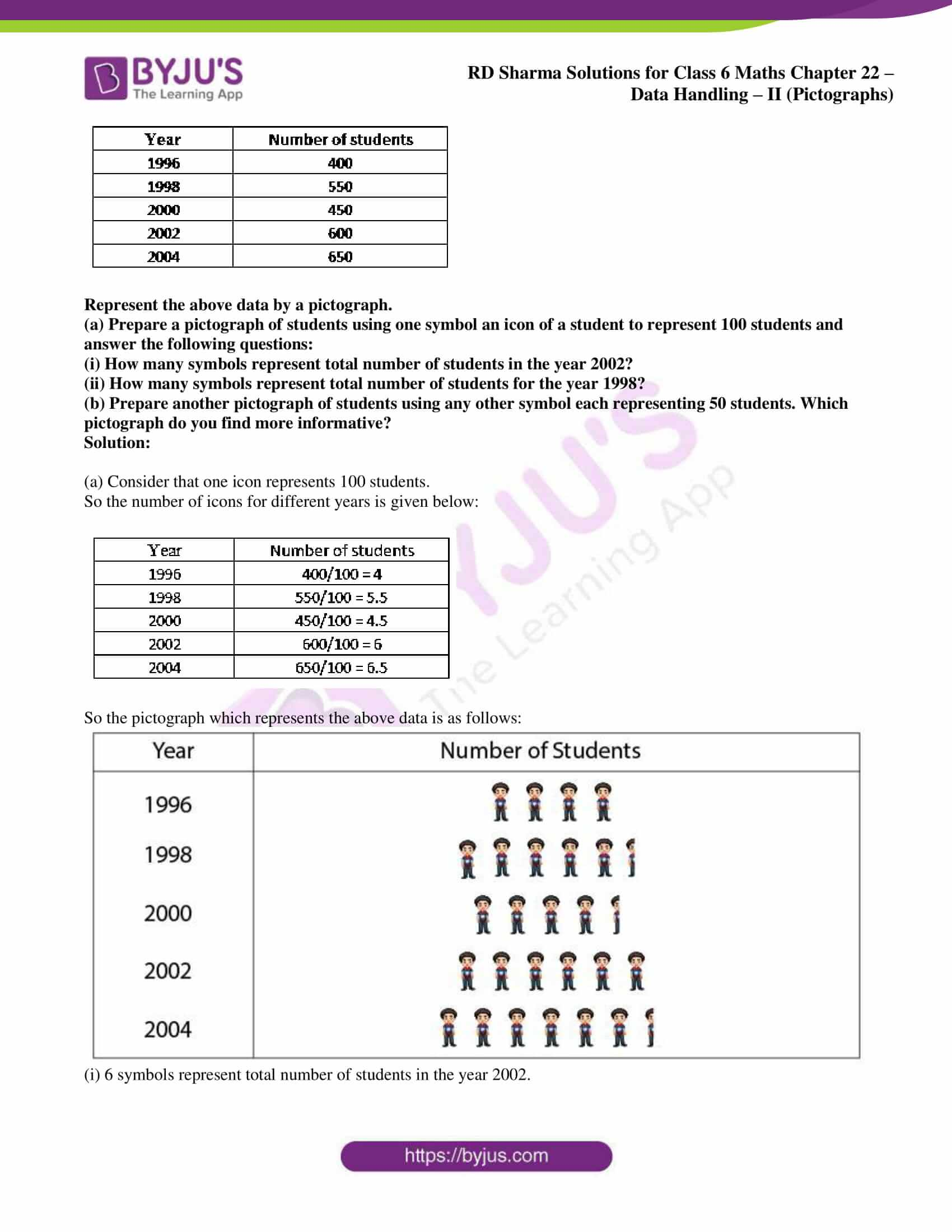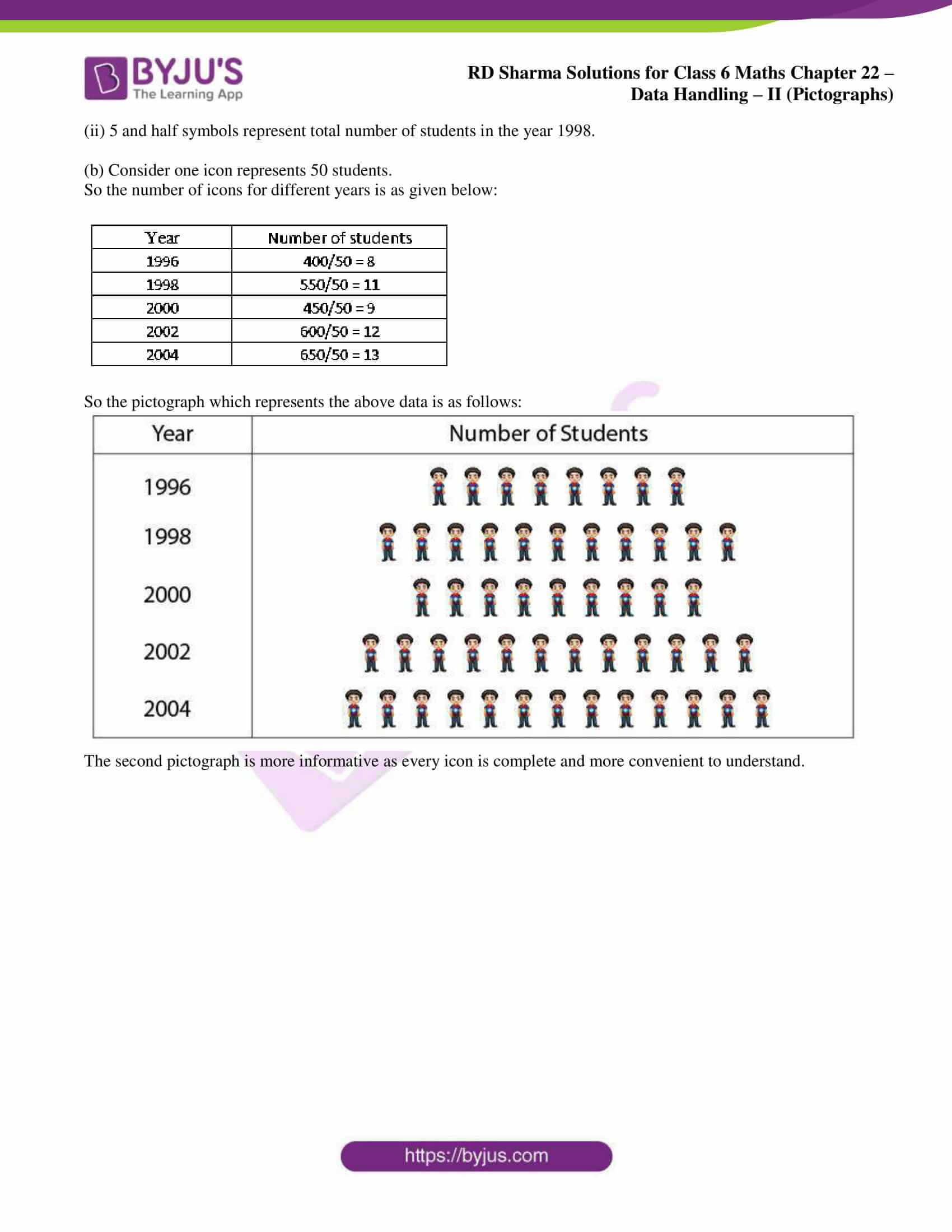## Access answers to Maths RD Sharma Solutions for Class 6 Chapter 22: Data Handling – II (Pictographs)

### Exercise 22.1 page: 22.5

1. The sale of electric bulbs on different days of a week is shown below: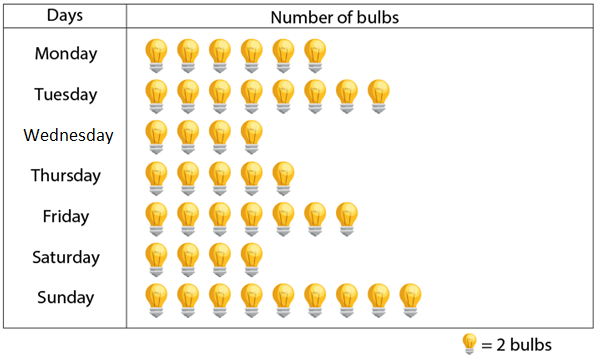Observe the pictograph and answer the following questions:

(i) How many bulbs were sold on Friday?

(ii) On which day maximum number of bulbs were sold?

(iii) If one bulb was sold at the rate of Rs 10, what was the total earning on Sunday?

(iv) Can you find out the total earning of the week?

(v) If one big carton can hold 9 bulbs. How many cartons were needed in the given week, more than 7, more than 8 or more than 9?

Solution:

(i) No. of bulbs shown on Friday = 7

Given that 1 figure = 2 bulbs

So the total number of bulbs sold on Friday = 2 × 7 = 14

Hence, 14 bulbs were sold on Friday.

(ii) From the pictograph, Sunday shows the maximum bulb figures.

Hence, maximum number of bulbs were sold on Sunday.

(iii) No. of bulb figures sold on Sunday = 2 × 9 = 18

Given that Cost of one bulb = Rs 10

So the total earning on Sunday = 10 × 18 = Rs 180

(iv) Total bulb figures shown throughout the week = 6 + 8 + 4 + 5 + 7 + 4 + 9 = 43

So the total number of bulbs = 43 × 2 = 86

Total earning of the week = 10 × 86 = Rs 860

(v) We know that total number of bulbs = 86

It is given that one carton can hold 9 bulbs

So for holding 86 bulbs we require = 86 × 9 = 774 cartons

Hence, more than 9 cartons are needed.

2. A survey was carried out in a certain school to find about the different modes of transport used by students to travel to school each day. 30 students of class VI were interviewed and the data obtained was displayed in the form of a pictographs given below: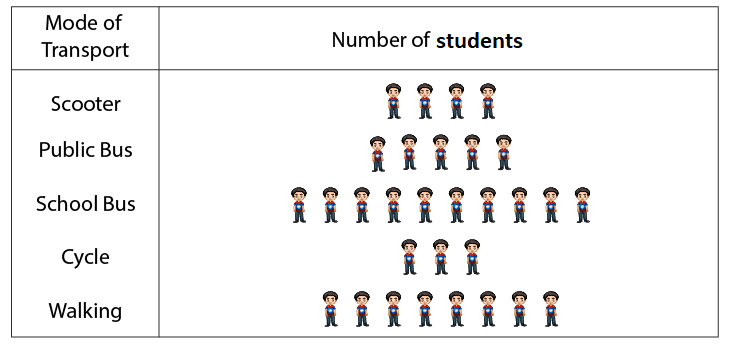Look at the above pictograph and answer the following questions:

(i) How many students are using cycle or walking as a mode of travel?

(ii) Which is most popular mode of travel?

Solution:

(i) From the pictograph we know that

No. of students using cycle = 3

No. of students using walking as a mode of travel = 8

So the number of students using cycle or walking as a mode of travel = 3 + 8 = 11

(ii) From the pictograph we know that maximum number of students use school bus as a mode of travel.

Hence, the most popular mode of travel to school is school bus.

3. The number of girl students in each class of co-ed. Middle school is depicted by the following pictograph: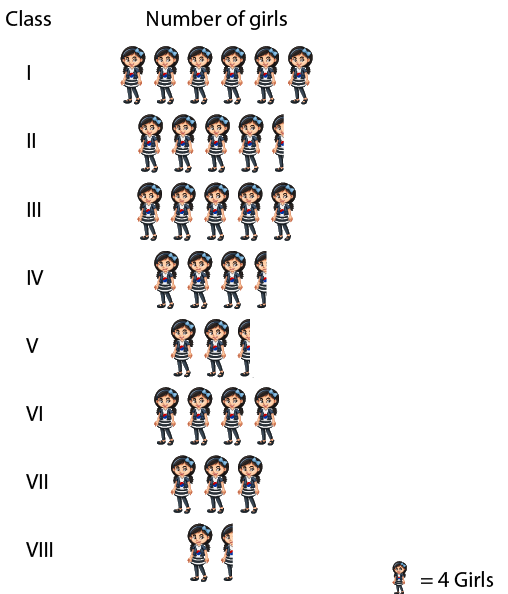Observe the above pictograph and answer the following questions:

(i) Which class has the maximum number of girl students?

(ii) Is the number of girls in class VI is less than the number of girls in class V?

(iii) How many girls are there in VII class?

Solution:

Using the above pictograph, we can prepare the chart to find the number of girl students in each class.

It is given that 1 figure = 4 girls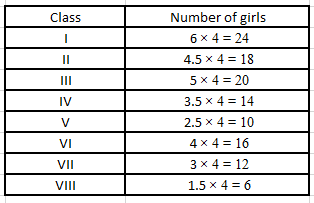(i) Using the chart we come to know that maximum number of girls are present in Class I.

Hence, Class I has the maximum number of girls.

(ii) Using the chart we come to know that

No. of girls in Class VI = 16

No. of girls in Class V = 10

No, the number of girls in class VI is not less than the number of girls in class V.

(iii) Using the chart we know that 12 girls are there in Class VII.

4. In a village six fruit merchants sold the following number of fruit baskets in particular season: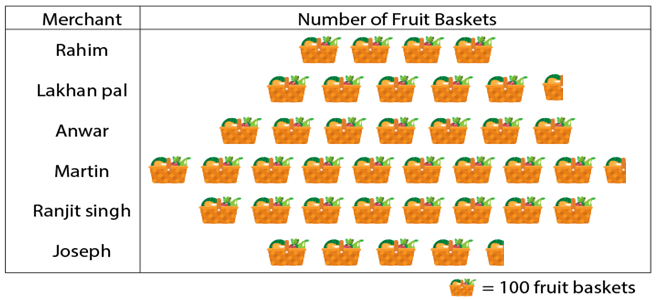Observe the above pictograph and answer the following questions.

(i) Which merchant sold the maximum number of baskets?

(ii) How many fruit baskets were sold by Anwar?

(iii) The merchants who have sold 600 or more number of baskets are planning to buy a godown for the next season. Can you name them?

Solution:

We know that 1 figure = 100 fruit baskets

Using the above pictograph, we can prepare a chart to find the maximum number of fruit baskets sold by each merchant.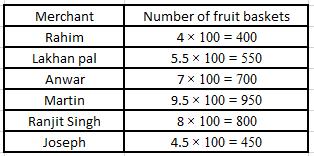(i) From the above chart we come to know that Martin has sold maximum number of fruit baskets.

(ii) 700 fruit baskets were sold by Anwar.

(iii) From the chart we know that Anwar has sold 700 fruit baskets, Martin has sold 950 fruit baskets and Ranjit singh has sold 800 fruit baskets.

Hence, Anwar, Martin and Ranjit Singh are planning to buy a godown for the next season.

5. The pictograph shows different subject books which are kept in a library.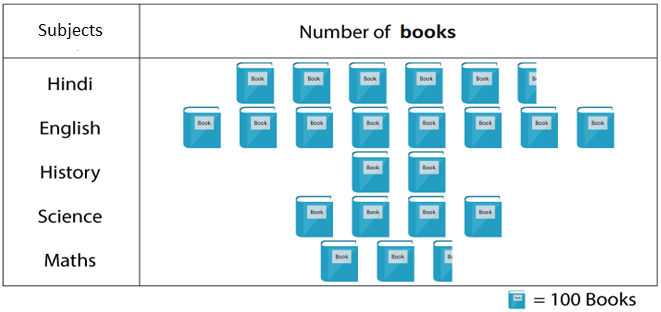(i) How many English books are there in the library?

(ii) How many Maths books are there?

(iii) Which books are maximum in number?

(iv) Which books are minimum in number?

Solution:

Using the above pictograph we can prepare the chart to find the number of books present in the library: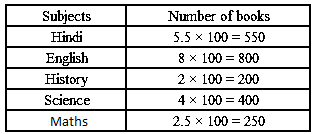(i) Using the chart, we come to know that 800 English books are there in the library.

(ii) From the chart, we come to know that 250 Math books are there in the library.

(iii) We come to know that English books are maximum in number from the above chart.

(iv) We come to know that History books are minimum in number from the above chart.

### Exercise 22.2 page: 22.9

1. The following are the details or the number of students in a class of 30 students present during a week.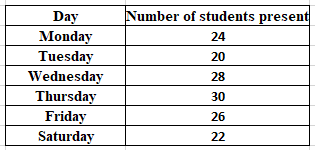Represent the above data by a pictograph.

Solution:

Consider that an icon of a student represent 4 students. So the number of icons for each day is given below: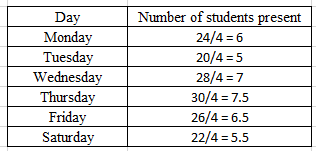So the pictograph which represents the above data is as follows: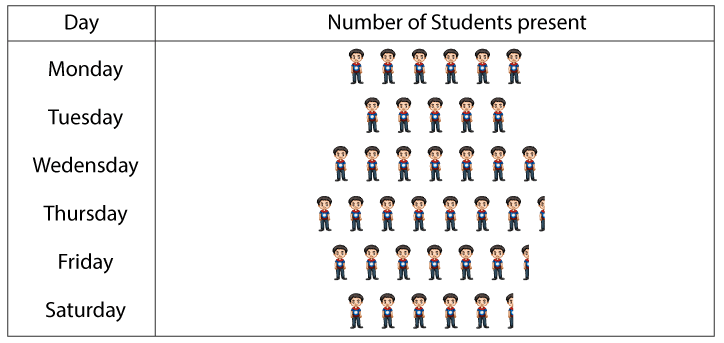2. Total number of students of a school in different years is shown in the following table: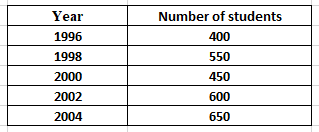Represent the above data by a pictograph.

(a) Prepare a pictograph of students using one symbol an icon of a student to represent 100 students and answer the following questions:

(i) How many symbols represent total number of students in the year 2002?

(ii) How many symbols represent total number of students for the year 1998?

(b) Prepare another pictograph of students using any other symbol each representing 50 students. Which pictograph do you find more informative?

Solution:

(a) Consider that one icon represents 100 students.

So the number of icons for different years is given below: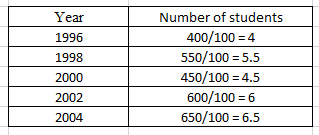So the pictograph which represents the above data is as follows: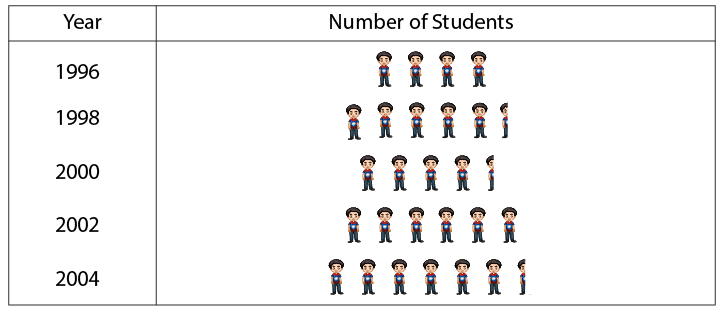(i) 6 symbols represent total number of students in the year 2002.

(ii) 5 and half symbols represent total number of students in the year 1998.

(b) Consider one icon represents 50 students.

So the number of icons for different years is as given below: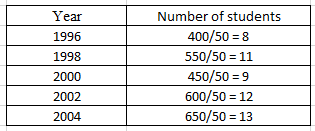So the pictograph which represents the above data is as follows: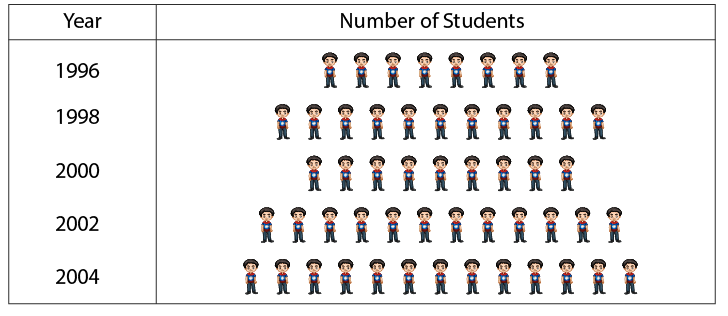The second pictograph is more informative as every icon is complete and more convenient to understand.